# How to Calculate and Solve for Flexural Strength for Circular Cross-section in Defects | Ceramics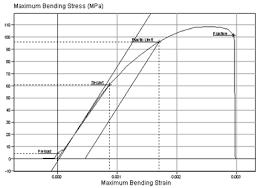The image above represents flexural strength for circular cross-section in defects.

To compute for flexural strength for circular cross-section in defects, three essential parameters are needed and these parameters are Load at fracture (Ff), Specimen radius (R) and Distance between support Points (L).

The formula for calculating flexural strength for circular cross-section in defects:

σfs = FfL / πR³

Where:

σfs = Flexural Strength
L = Distance between Support Points

Lets’s solve an example;
Find the flexural strength when the distance between support points is 30, load at fracture is 21 and the specimen radius is 11.

This implies that;

L = Distance between Support Points = 30
Ff = Load at Fracture = 21
R = Specimen Radius = 11

σfs = FfL / πR³
σfs = (21)(30) / π(11)³
σfs = (630) / π(1331)
σfs = (630) / (4181.4)
σfs = 0.150

Therefore, the flexural strength for circular cross-section is 0.150 Pa.

Calculating the Distance Between Support Points when the Flexural Strength for Circular Cross-section, the Specimen Radius and the Load at Fracture is Given.

L = σfs x πR³ / Ff

Where;

L = Distance between Support Points
σfs = Flexural Strength

Let’s solve an example;
Find the distance between support points when the flexural strength is 44, load at fracture is 3 and specimen radius is 20.

This implies that;

σfs = Flexural Strength = 44
Ff = Load at Fracture = 3
R = Specimen Radius = 20

L = σfs x πR³ / Ff
L = 44 x π x 3³ / 20
L = 44 x π x 27 / 20
L = 3732.2 / 20
L = 186.61

Therefore, the distance between support point is 186.61.

Calculating the Load at Fracture when the Flexural Strength, the Specimen Radius and the Distance Between Support Point is Given.

Ff = σfs x πR³ / L

Where;

σfs = Flexural Strength
L = Distance between Support Points

Let’s solve an example;
Find the load at fracture when the distance between support points is 14, the flexural strength is 7 and the specimen radius is 4.

This implies that;

σfs = Flexural Strength = 7
L = Distance between Support Points = 14
R = Specimen Radius = 4

Ff = σfs x πR³ / L
Ff = 7 x π x 4³ / 14
Ff = 7 x π x 64 / 14
Ff = 1407.4 / 14
Ff = 100.5

Therefore, the load at fracture is 100.5.

Calculating the Specimen Radius when the Flexural Strength, the Distance Between Support Points and the Load at fracture is Given.

R = 3√(FfL / σfs x 1 / π)

Where;

σfs = Flexural Strength
L = Distance between Support Points

Let’s solve an example;
Find the specimen radius when the flexural strength is 18, the distance between support points is 11 and the load at fracture is 7.

This implies that;

σfs = Flexural Strength = 18
L = Distance between Support Points = 11
Ff = Load at Fracture = 7

R = 3√(FfL / σfs x 1 / π)
R = 3√(7 x 11 / 18 x 1 / π)
R = 3√(77 / 18 x 0.318)
R = 3√(4.27 x 0.318)
R = 3√1.35786
R = 1.107

Therefore, the specimen radius is 1.107.

Nickzom Calculator – The Calculator Encyclopedia is capable of calculating the flexural strength for circular cross-section.

To get the answer and workings of the flexural strength for circular cross-section using the Nickzom Calculator – The Calculator Encyclopedia. First, you need to obtain the app.

You can get this app via any of these means:

To get access to the professional version via web, you need to register and subscribe for NGN 1,500 per annum to have utter access to all functionalities.
You can also try the demo version via https://www.nickzom.org/calculator

Apple (Paid) – https://itunes.apple.com/us/app/nickzom-calculator/id1331162702?mt=8
Once, you have obtained the calculator encyclopedia app, proceed to the Calculator Map, then click on Materials & Metallurgical under Engineering.Now, Click on Ceramics under Material & MetallurgicalNow, Click on Flexural Strength for Circular Cross-Section in Defects under Ceramics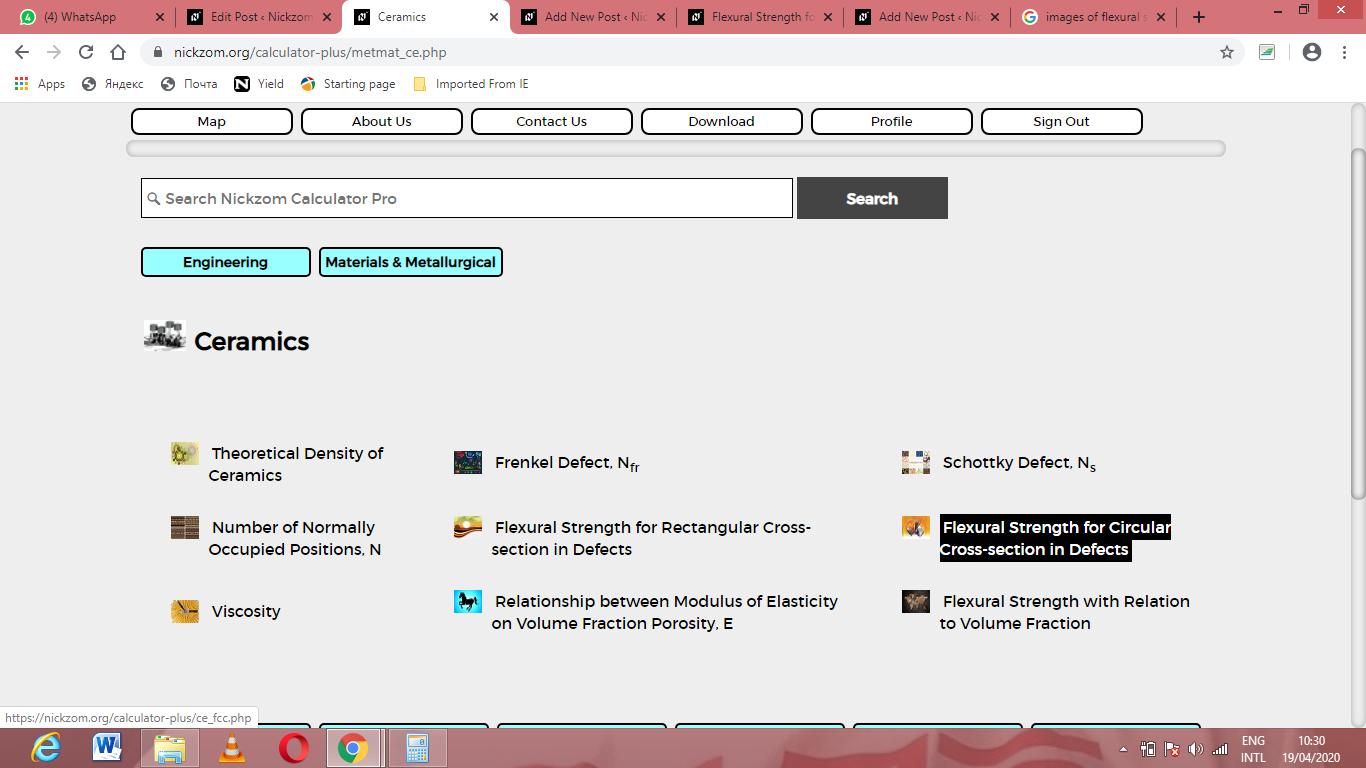The screenshot below displays the page or activity to enter your values, to get the answer for the flexural strength for circular cross-section in defects  according to the respective parameters which are the Load at fracture (Ff), Specimen radius (R) and Distance between support Points (L).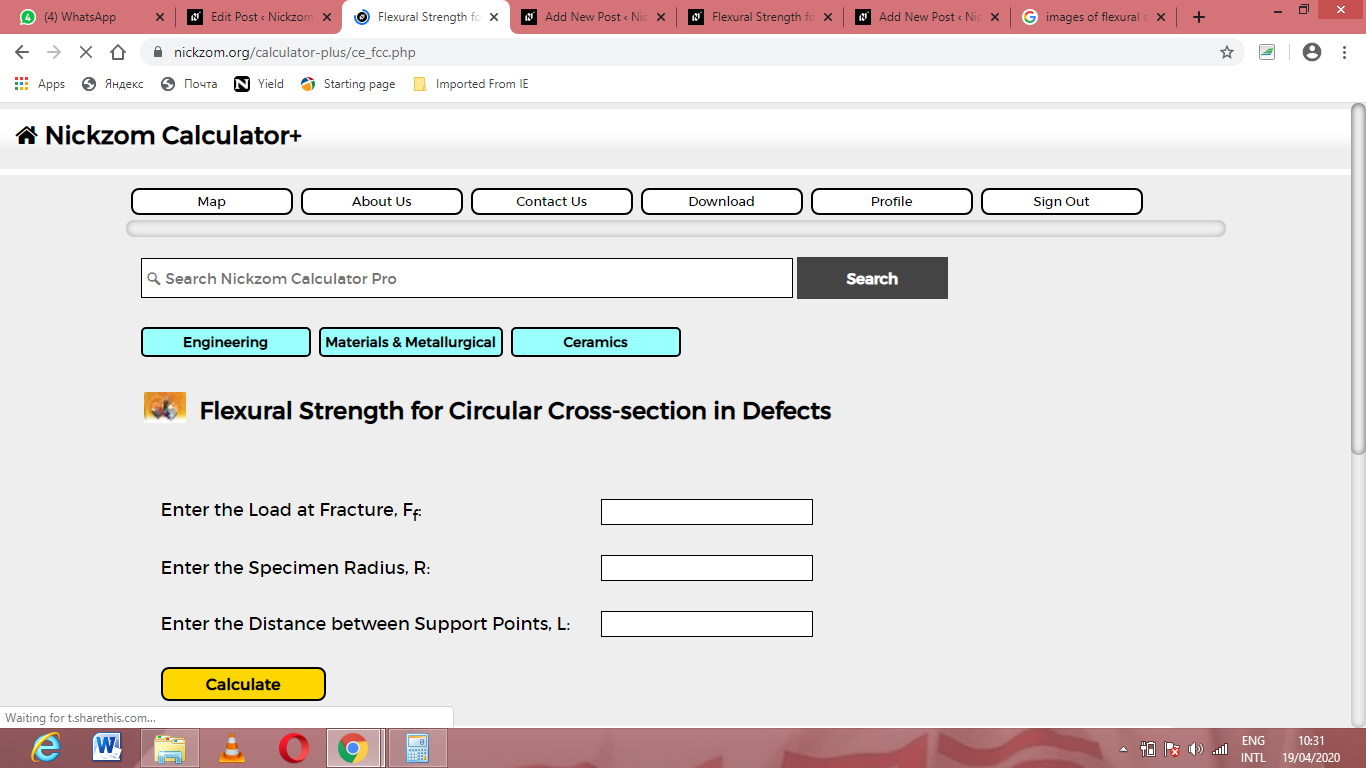Now, enter the values appropriately and accordingly for the parameters as required by the Load at fracture (Ff) is 21, Specimen radius (R) is 11 and Distance between support Points (L) is 30.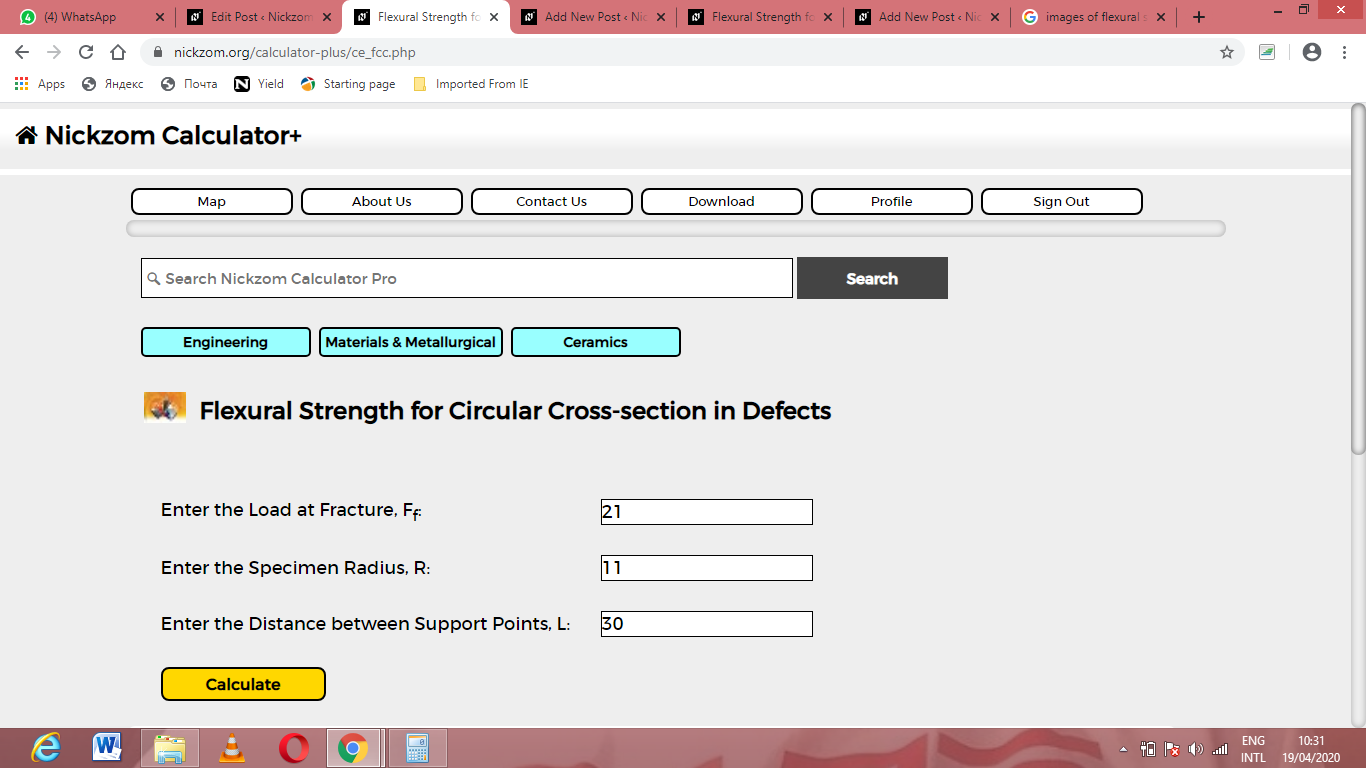Finally, Click on Calculate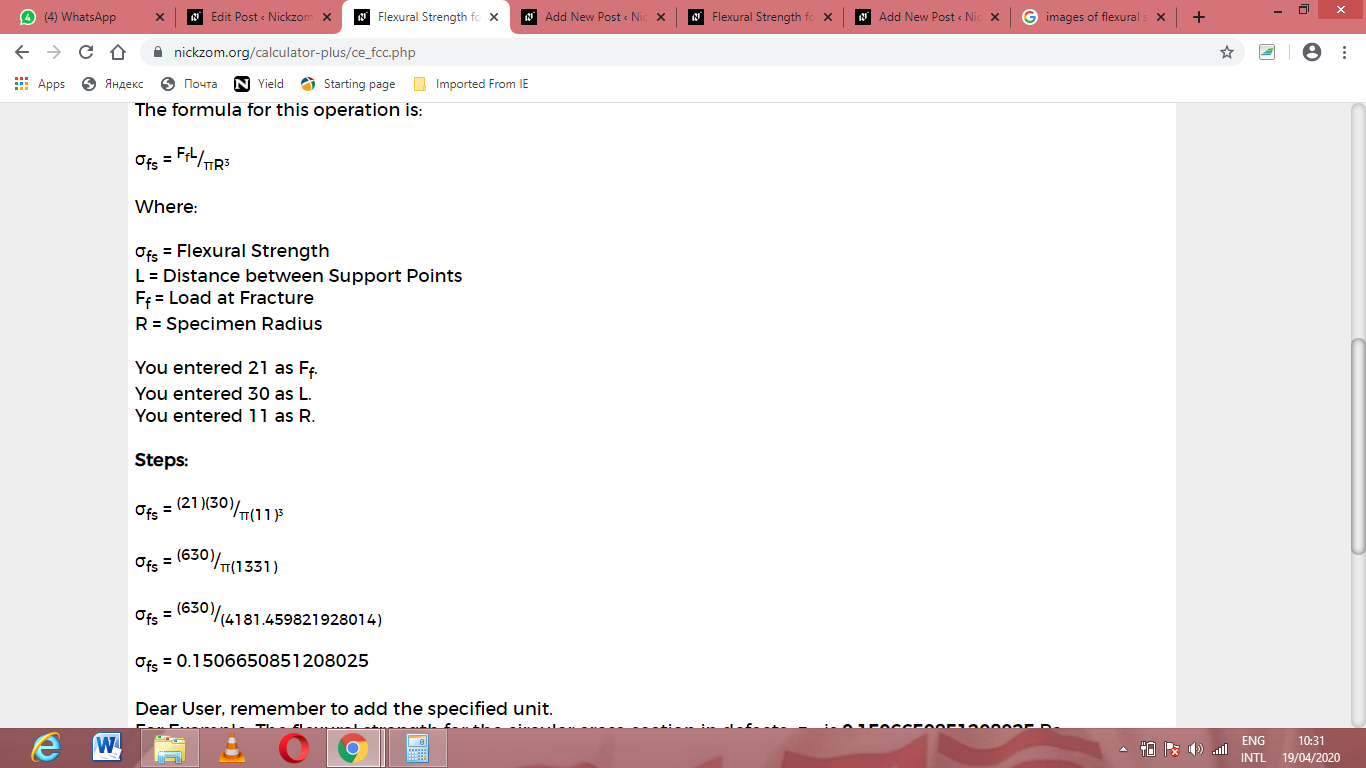As you can see from the screenshot above, Nickzom Calculator– The Calculator Encyclopedia solves for the flexural strength for cross-section in defects and presents the formula, workings and steps too.